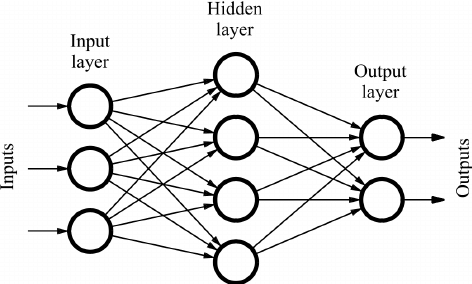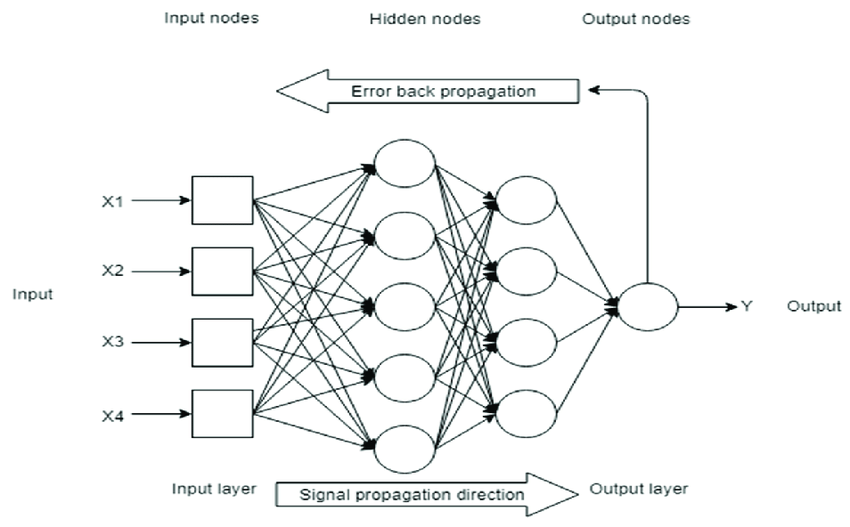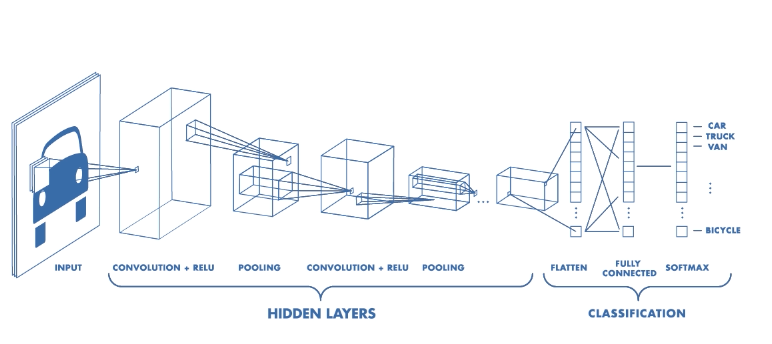×

Search anything:

# Deep Learning mock interview

#### Deep Learning List of Interview QuestionsGet this book -> Problems on Array: For Interviews and Competitive Programming

In this article at OpenGenus, we will understand the flow of a deep learning interview for a data science based role.

Deep learning is another essential skill needed to bag the most sought after job role of the century - "Data Scientist". It is comparitively difficult to work with than normal machine learning and hence requires a strong foundation to get the hang of it. A deep learning interview round is conducted to test our skills in the field and is the round where the interviewer get to grill us out - the questions may get a bit tough. But if we have good knowledge and understanding of the required concepts, this is just another interview round!

## Interviewer: What is the difference between Machine Learning and Deep Learning?

Machine learning Deep learning
It is the superset of deep learning. It is the subset if machine learning.
More human intervention is needed for getting results. Once setup, it requires less human intervention.
Feature engineering is explicitly done by humans. Feature engineering is not needed.
ML applications are comparitively simpler. Deep learning models use powerful hardware and resources.
Uses various types of algorithms to solve problems. Makes use of neural networks.

## Interviewer: Do you think deep learning is better than machine learning? If so, why?

Yes, deep learning is better than machine learning as ML algorithms are not useful when working with high-dimensional data. The second drawback of ML is that it requires us to specify the features that it should consider for predicting the outcome.

## Interviewer: What is a perceptron?

Similar to a biological neuron that has dendrites to receive inputs, a perceptron is a linear model used for binary classification that receives multiple inputs, applies various transformations and functions and gives an output. Here, each of the input has a specified weight.

## Interviewer: What are the drawbacks of a single layer perceptron?

The two major drawbacks of a single layer perceptron are:

• It cannot classify data points that have non-linear boundaries.
• Complex problems that include a lot of parameters cannot be solved by a single layer perceptron.

## Interviewer: Name the different components of a multilayer perceptron.

The three components of a multilayer perceptron are:

• Input layer
• Hidden layers
• Output layer

## Interviewer: Can you build a basic multilayer perceptron model?

``````import tensorflow as tf
import numpy as np
from tensorflow.keras.models import Sequential
from tensorflow.keras.layers import Flatten
from tensorflow.keras.layers import Dense
from tensorflow.keras.layers import Activation
import matplotlib.pyplot as plt

(x_train, y_train), (x_test, y_test) = tf.keras.datasets.mnist.load_data()

x_train = x_train.astype('float32')
x_test = x_test.astype('float32')
gray_scale = 255
x_train /= gray_scale
x_test /= gray_scale

model = Sequential([
Flatten(input_shape=(28, 28)),
Dense(256, activation='sigmoid'),
Dense(128, activation='sigmoid'),
Dense(10, activation='sigmoid'),
])
loss='sparse_categorical_crossentropy',
metrics=['accuracy'])
model.fit(x_train, y_train, epochs=15,
batch_size=2000,
validation_split=0.2)
``````

## Interviewer: In the above program, you have used 'Adam' optimizer. Can you tell me about it?

Instead of stochastic gradient descent, adam is used as an optimization algorithm in the above program. Adam combines the advantages of Adaptive Gradient Algorithm and Root Mean Square Propagation to handle sparse gradients on noisy problems. It is relatively easy to configure and has four configuration parameters - alpha, beta1, beta2 and epsilon.

## Interviewer: What is a cost function?

A cost function is the measure of accuracy of the neural network with respect to a given sample and output. It provides the performance of the neural network as a whole. The main aim in deep learning is to minimize the cost function. This is done using gradient descent.

## Interviewer: Can you tell the steps for gradient descent?

The steps for gradient descent algorithm are as follows.

1. Initialize random weight and bias.
2. Pass an input through the network and get the output value.
3. Find the error between the actual and predicted value.
4. Reduce the values of respective neurons that cause the error.
5. Keep on iterating until we find the best weights for the network.

## Interviewer: What are the different variants of gradient descent?

There are three different variants of gradient descent. They are:

## Interviewer: Why is mini-batch gradient descent so popular?

Mini-batch gradient descent is more efficient compared to stochastic gradient descent and here, generalization is done by finding the flat minima. One more advantage is that it allows approximation of gradient to the whole dataset thus helping to avoid the local minima. These are the reasons why this optimization algorithm is so popular.

## Interviewer: What is the difference between an feed-forward and back propagation neural network?

A feed forward neural network is the type of neural network architecture where connections are "fed-forward". They do not form cycles.A back propagation algorithm consists of two main steps:

• Feed forward the values
• Calculate the error and propagate it back to the layers before.## Interviewer: What do you understand by convolutional neural networks?

Convolutional neural networks are mostly applied to analyzing visual imagery and are a class of deep neural networks. Here, input is a multi-channeled image unlike the vector input in neural networks. CNN uses a variation of multi-layer perceptron that are designed to require minimal pre-processing.## Interviewer: Explain the different layers of convolutional neural networks.

There are four main layers in the convolutional neural network architecture. They are:

• Convolution - This layer comprises of a set of independent filters.
• ReLu - This is the Rectified Linear Unit layer used with the convolution layer.
• Pooling - Its function is to progressively reduce the number of parameters and computations in the network, along with reducing the spatial size of the representation.
• Fully-connected layer - Neurons in the fully connected layer have connections to all activations in the previous layer.

## Interviewer: What is a restricted Boltzmann machine?

Restricted Boltzmann machine is an undirected graphical model that plays a major role in deep learning framework in recent times. It is an algorithm which is useful for dimensionality reduction, classification, regression, collaborative filtering, feature learning and topic modeling.

Interview: That's all for this interview. HR will convey the feedback and provide you with the next steps. Best of luck.#### Sanjana Babu

Sanjana Babu is a Student at SRM Valliammai Engineering College and is an Intern at OpenGenus. She is a Philomath.